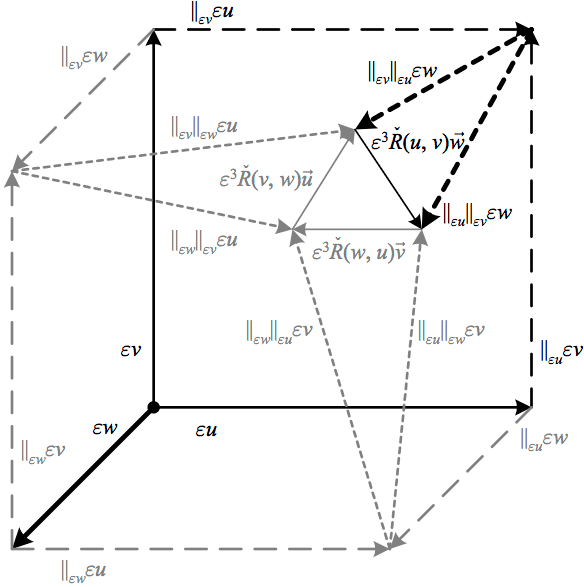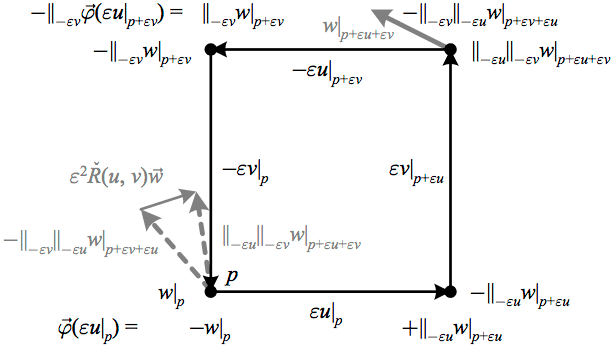# Intuitive explanation for Riemann tensor definition

Many sources give explanations of the Riemann tensor that involve parallel transporting a vector around a loop and finding its deviation when it returns. They then show that this same tensor can be derived by taking the commutator of second covariant derivatives. Is there a way to understand why these two derivations are related? In other words, is there an intuitive way to get to the commutator definition of the Riemann tensor directly from the idea of parallel transport?

You can start with the definition of the Riemann tensor which uses the commutator, and then you parallel transport two vectors to find that they differ at the end point, and notice that the difference is exactly the same as the riemann tensor as you defined using the commutator (aside from some other terms present in the result).

Orodruin
Staff Emeritus
Homework Helper
Gold Member
2021 Award
The covariant derivative of a field in a direction ##X## measures the change in the field relative to a parallel transported field if you go in direction ##X##. Hence, ##\nabla_X \nabla_Y Z## is a measure of the change in ##\nabla_Y Z## relative to a parallel transport when you go in direction ##X##, i.e., the change of the change of ##Z## relative to a parallel transport in direction ##Y## relative to a parallel transport in direction ##X## (there are lots of "change" because it is a second derivative ... but ultimately it is a measure of how ##Z## changes to leading order since the first derivatives will cancel out). Hence, you have the change relative to parallel transport along ##Y## first and then ##X## compared to the change relative to parallel transport along ##X## first and then ##Y##. Since ##Z## is the same vector field in both cases, the difference between those gives you the difference between the two parallel transports.

Now, the above is true as long as ##[X,Y] = 0##. When ##[X,Y] \neq 0## then the flows along ##X## and ##Y## do not commute and therefore the loop does not close and you need to compensate by also noting how ##Z## changes relative to a parallel transport of ##Z## along ##[X,Y]##. You therefore end up with
$$R(X,Y)Z = \nabla_X \nabla_Y Z - \nabla_Y \nabla_X Z - \nabla_{[X,Y]} Z$$
as a measure of how a vector ##Z## would change upon parallel transport around an infinitesimal loop spanned by ##X## and ##Y##.

You can start with the definition of the Riemann tensor which uses the commutator, and then you parallel transport two vectors to find that they differ at the end point, and notice that the difference is exactly the same as the riemann tensor as you defined using the commutator (aside from some other terms present in the result).
While this is true (and it is how I do it in my book), I do not believe that it answers the OP's question. The question was whether you can produce an intuitive way of obtaining the definition of the curvature tensor in terms of the connection just from considering parallel transport, not if you could get the "change after parallel transport around a loop" interpretation from the definition in terms of the connection).

Last edited:
•strangerep and vanhees71
Parallel displacement of vectors to a destination but in different order, e.g. first dx then dy vs first dy then dx, Do not coincide. Difference by route gives R.

We can also choose start and goal a same point and ratio of difference divided by area of closed loop gives R.

Last edited:
pervect
Staff Emeritus
Many sources give explanations of the Riemann tensor that involve parallel transporting a vector around a loop and finding its deviation when it returns. They then show that this same tensor can be derived by taking the commutator of second covariant derivatives. Is there a way to understand why these two derivations are related? In other words, is there an intuitive way to get to the commutator definition of the Riemann tensor directly from the idea of parallel transport?

Pick three points. Three points determine a plane. If the three points are close enough, there is one and only one geodesic connecting any pair of the points, so we form a unique geodesic triangle by picking three points. (The region where this is true is called something like the local convex region, I believe).

The next step in the intuitive picture is to relate the sum of angles of the triangle to the process of parallel transport. We are aided by the fact that a geodesic, by definition, parallel transports a vector along itself. And in GR, we can take advantage of the metric compatibility condition that says that if we parallel transport two (or more) vectors along a curve, the angle between the vectors doesn't change. (This might not be true in a non-GR context).

I believe it's possible to convince oneself by drawing some diagrams to relate the sum of the exterior angle of the triangles to the amount of rotation of a vector parallel transported around the geodesic triangle. But I haven't drawn up the necessary figures to make a solid argument for it. Basically, I start with a vector tangent to the first side of the geodesic triangle, parallel tranport this vector to the next vertex of the triangle, add in a second vector representing the second side, parallel tranporting both of those along the second side, add in a third vector at the third vertex, then transport all three vectors back to the starting point.

One then also needs to relate the sum of exterior angles of the triangle to the more usual some of interior angles - this is easy, the tricky part is convincing onself about the sum of the exterior angles.

This is convenient for intuitive explanations , because it's easy to find a lot of theorems about the sum-of-interior-angles in spehrical trignometry, and how they relate to the area of the triangle. See for instance wiki.

Orodruin
Staff Emeritus
Homework Helper
Gold Member
2021 Award
And in GR, we can take advantage of the metric compatibility condition that says that if we parallel transport two (or more) vectors along a curve, the angle between the vectors doesn't change.
This is true for any metric compatible connection as it is a direct consequence of the connection being metric compatible, i.e. ##\nabla_Z^{} g = 0## for all ##Z##. In particular, if ##X## and ##Y## are parallel along a curve ##\gamma##, then
$$\frac{d(g(X,Y))}{ds} = \nabla_{\dot\gamma} g(X,Y) = (\nabla_{\dot\gamma} g)(X,Y) + g(\nabla_{\dot\gamma} X,Y) + g(X,\nabla_{\dot\gamma} Y) = 0 + 0 + 0,$$
where ##\dot\gamma## is the tangent vector of the curve, the first term vanishes due to metric compatibility and the two latter due to ##X## and ##Y## being parallel transported along ##\gamma##. Hence, parallel transport using a metric compatible connection preserves the inner product between vectors. In GR we typically use the Levi-Civita connection, which apart from being metric compatible is also torsion free.

I believe it's possible to convince oneself by drawing some diagrams to relate the sum of the exterior angle of the triangles to the amount of rotation of a vector parallel transported around the geodesic triangle.
This is true only in two dimensions. When you are dealing with more than two dimensions you can rotate around the tangent vector of the geodesics in addition to the fixed angle relative to the geodesic, which will generally change the final rotation.

I also do not see how these statements, or the one in #4, give an answer to the question in the OP:
Is there a way to understand why these two derivations are related? In other words, is there an intuitive way to get to the commutator definition of the Riemann tensor directly from the idea of parallel transport?
I.e., the OP wants to know why the definition of the Riemann tensor in terms of the connection
$$R(X,Y)Z = \nabla_X \nabla_Y Z - \nabla _Y \nabla_X Z - \nabla_{[X,Y]} Z$$
is intuitively related to the change in ##Z## when parallel transported around a loop. Any explanation of that must start from the interpretation of ##\nabla_X Z## being the difference between ##Z## at a nearby point and the vector you would obtain by parallel transporting ##Z## from the original point. In addition, the definition of the Riemann tensor, and therefore also its geometrical interpretation, is completely independent of the existence of a metric as it only relates to the connection that is imposed on the manifold.

•strangerep and vanhees71
pervect
Staff Emeritus
I see the notation R(X,Y)Z used all the time, for instance in wiki, but it's not a notation that my phsyics textbooks (Wald, MTW) ever use. I suspect it's a notational difference between mathematicians and physicists.

In abstract index notation, would R(X,Y)Z be ##R^a{}_{zxy}##? A map from three vectors (X,Y,Z) to another vector (##R^a## in abstract index notation)? With X and Y being anti-symmetric?

Orodruin
Staff Emeritus
Homework Helper
Gold Member
2021 Award
I see the notation R(X,Y)Z used all the time, for instance in wiki, but it's not a notation that my phsyics textbooks (Wald, MTW) ever use. I suspect it's a notational difference between mathematicians and physicists.

In abstract index notation, would R(X,Y)Z be ##R^a{}_{zxy}##? A map from three vectors (X,Y,Z) to another vector (##R^a## in abstract index notation)? With X and Y being anti-symmetric?
If just talking about the tensor itself, I would just write ##R##, although that would have possible notational issues if you also denote the Ricci scalar by ##R##. You can avoid that by typesetting the Ricci scalar differently, e.g., ##\mathcal R##. ##R(X,Y)Z## is actually a tangent vector and in abstract index notation would be written ##R_{bcd}^a X^c Y^d Z^b##. ##R(X,Y)Z## is indeed anti-symmetric under ##X \leftrightarrow Y##. I think one should be aware of both notations, but although I am a physicist I actually prefer using index-free notation as much as reasonably possible. The actual components of the Riemann tensor would be given by
$$R(e_b,e_c)e_a = R^d_{abc} e_d,$$
where ##e_a## is the chosen basis.

It is actually not too difficult to directly get to the commutator definition of the Riemann tensor from the idea of parallel transport. For infinitesimal parallel transport from a point ##p## along a curve ##\varepsilon v## with tangent ##v##, the covariant derivative is defined as
$$\nabla_{v}w\equiv\underset{\varepsilon\rightarrow0}{\textrm{lim}}\frac{1}{\varepsilon}\left(w\left|_{p+\varepsilon v}\right.-\parallel_{\varepsilon v}\left(w\left|_{p}\right.\right)\right),$$
so dropping the limit,
$$\parallel_{\varepsilon v}(w\left|_{p}\right.)=w\left|_{p+\varepsilon v}\right.-\varepsilon\nabla_{v}w\left|_{p}\right..$$
Applying twice, we have
\begin{aligned}\parallel_{\varepsilon u}\parallel_{\varepsilon v}(w\left|_{p}\right.) & =\parallel_{\varepsilon u}\left(w\left|_{p+\varepsilon v}\right.-\varepsilon\nabla_{v}w\left|_{p}\right.\right)\\ & =w\left|_{p+\varepsilon v+\varepsilon u}\right.-\varepsilon\nabla_{v}w\left|_{p+\varepsilon u}-\varepsilon\nabla_{u}w\left|_{p+\varepsilon v}\right.+\varepsilon^{2}\nabla_{u}\nabla_{v}w\left|_{p},\right.\right., \end{aligned}
so that
\begin{aligned}\parallel_{\varepsilon u}\parallel_{\varepsilon v}(w\left|_{p}\right.)-\parallel_{\varepsilon v}\parallel_{\varepsilon u}(w\left|_{p}\right.) & =\varepsilon^{2}\nabla_{u}\nabla_{v}w\left|_{p}\right.-\varepsilon^{2}\nabla_{v}\nabla_{u}w\left|_{p}\right.\\ & =\varepsilon^{2}\check{R}(u,v)\vec{w}. \end{aligned}
Here we have assumed ##[u,v]=0##, so that ##w\left|_{p+\varepsilon v+\varepsilon u}\right.=w\left|_{p+\varepsilon u+\varepsilon v}\right.##. This actually doesn’t constrict the result, since the curvature being a tensor means that ##\check{R}(u,v)\vec{w}## only depends upon the local values of ##u## and ##v##, so we are free to construct their vector field values such that ##[u,v]=0##.

This approach, detailed here, can be used to build a geometric picture of the first Bianchi identity:One can also start from the definition in terms of the exterior covariant derivative ##\check{R}\left(u,v\right)\vec{w}=\left(\mathrm{D}^{2}\vec{w}\right)(u,v)## to arrive at another picture in terms of parallel transport:More details here.

#### Attachments

•strangerep